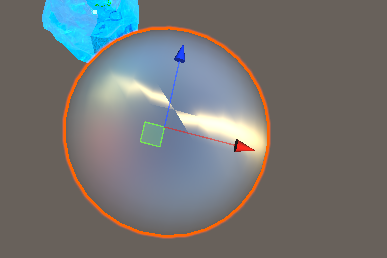# GGX Anisotropy BRDF

Good Morning.
I am implementing a BRDF anisotropy model.
However it was strange.

GGX BRDF

`````` //Anisotropy Surfaces
float SpecularStrengthAnisotropy(Surface surface, BRDF brdf, Light light) {
float at = max(brdf.roughness * (1.0 + surface.anisotropic), 0.001);
float ab = max(brdf.roughness * (1.0 - surface.anisotropic), 0.001);

float3 h = SafeNormalize(light.direction + surface.viewDirection);
float NoH = dot(surface.normal, h);
float ToH = dot(surface.tangent, h);

float3 b = normalize(cross(surface.normal, surface.tangent));//normalize(cross(surface.normal, surface.tangent.xyz) * surface.tangent.w);

float BoH = dot(b, h);
float a2 = at * ab;
float3 v = float3(ab * ToH, at * BoH, a2 * NoH);
float v2 = dot(v, v);
float w2 = a2 / v2;
return a2 * w2 * w2 * (1.0 / PI);
}
``````

ResultWhere did I go wrong?
How can I fix this?

I don’t know this brdf, but this looks strange to me:

This topic was automatically closed 183 days after the last reply. New replies are no longer allowed.